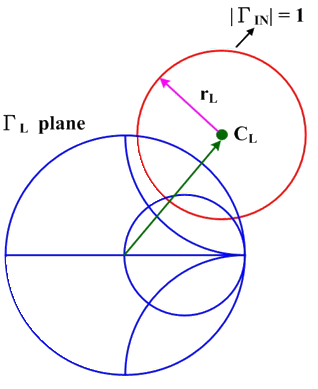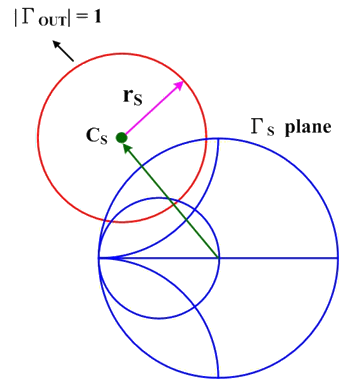Module 10.2 Stability Circle of Transistor
|

 Freq (GHz) MagS11 (Magnitude) AngS11 (Phase Angle) MagS21 (Magnitude) AngS21 (Phase Angle) MagS12 (Magnitude) AngS12 (Phase Angle) MagS22 (Magnitude) AngS22 (Phase Angle)

Simultaneous conjugate match conditions

Γ_(MS)(Magnitude, Phase Angle) =

Γ_(ML)(Magnitude, Phase Angle) =

Make a common Sparameter.s2p file.
Step 1 : the Sparameter.s2p template file from example.
Step 2 : Open the Sparameter.s2p with a text editor.
Step 3 : Replace the value of the Freq., Mag., and Ang. with your device Sparameter
Step 4 : Save the file and exit.
Step 5 : Browse and submit your new Sparameter.s2p file.

Introduction:

Stability circle is used to determine the stability of the two-port network:

### Output stability circle Γ_L values for |Γ_(IN)| = 1

|Γ_(IN)| = |S_(11)+(S_(21) S_(12) Γ_(L))/(1-S_(22) Γ_(L))| = 1

|Γ_(L) - (S_(22)-∆S_(11)^**)^**/(|S_(22)|^2-|∆|^2 )| = |(S_(12) S_(21))/(|S_(22) |^2-|∆|^2 )|

r_(L) = |(S_(12) S_(21))/(|S_(22) |^2-|∆|^2 )| (radius)

C_(L)= (S_22-∆S_(11)^**)^**/(|S_(22) |^2-|∆|^2 ) (center)

∆ = S_(11) S_(22)-S_(12) S_(21) = det [S]

### Input stability circle Γ_s values for |Γ_(OUT)| = 1

|Γ_(OUT)| = |S_(22) + (S_(21) S_(12) Γ_(S))/(1- S_(11) Γ_(S) )| = 1

|Γ_(S) - (S_(11)-∆S_(22)^**)^**/(|S_11 |^2-|∆|^2 )| = |(S_(12) S_(21))/(|S_(11) |^2-|∆|^2 )|

r_s = | (S_(12) S_(21)) / (|S_(11) |^2-|∆|^2 ) | (radius)

C_(S)= (S_(11)-∆S_(22)^**)^** / (|S_(11) |^2 - |∆|^2 ) (center)

∆ = S_(11) S_(22) - S_(12) S_(21) = det [S]r_(L) = The radius of the output stability circle c_(L) = The center of the output stability circle. r_(S) = The center of the input stability circle. c_(S) = The center of the input stability circle. Γ_(L) = The reflection coefficient of the output. Γ_(S) = The reflection coefficient of the input. Simultaneous conjugate match conditions.       The conditions required to obtain maximum transducer power gain: Γ_S = Γ_(IN)^**     Γ_L = Γ_(OUT)^** Γ_S^** = S_(11) + (S_(21) S_(12) Γ_(L))/(1- S_(22) Γ_(L)) Γ_L^** = S_(22) + (S_(21) S_(12) Γ_(S))/(1- S_(11) Γ_(S)) Γ_(ML) = The simultaneous conjugate match of the output. Γ_(MS) = The simultaneous conjugate match of the input. Γ_(MS) = (B_(1) ± sqrt((B_(1)) ^2 -4|C_(1)|^2 ))/(2C_(1)) Γ_(ML) = (B_(2) ± sqrt((B_(2)) ^2 -4|C_(2)|^2 ))/(2C_(2)) B_1 = 1+|S_11 |^2-|S_22|^2-|∆|^2 B_2 = 1+|S_22 |^2-|S_11 |^2-|∆|^2 C_1 = S_11-∆S_(22)^** C_2 = S_22-∆S_(11)^**